Next: Inference from a data Up: Inferring numerical values of Previous: Binomial model

## Poisson model

The Poisson distribution gives the probability of observing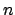counts in a fixed time interval, when the expectation of the number of counts to be observed is: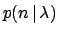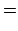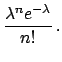(43)

The inverse problem is to inferfromcounts observed. (Note that what physically matters is the rate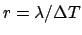, where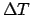is the observation time.) Applying Bayes' theorem and using a uniform prior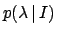for, we get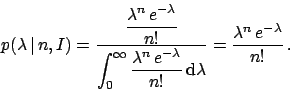(44)

As for the Gaussian model, the same mathematical expression holds for the likelihood, but with interchanged role of variable and parameter. Expectation and variance ofare both equal to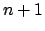, while the most probable value is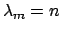. For large, the extra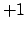' (due to the asymmetry of the prior with respect to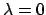) can be ignored and we have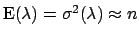and, once again, the uncertainty aboutfollows a Gaussian model. The relative uncertainty ondecreases as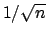.

When the observed value ofis zero, Eq. (44) yields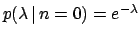, giving a maximum of belief at zero, but an exponential tail toward large values of. Expected value and standard deviation ofare both equal to 1. The 95% probabilistic upper bound ofis at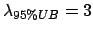, as it can be easily calculated solving the equation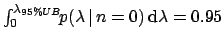. Note that also this result depends on the choice of prior, though Astone and D'Agostini (1999) have shown that the upper bound is insensitive to the exact form of the prior, if the prior models somehow what they call positive attitude of rational scientists'' (the prior has not to be in contradiction with what one could actually observe, given the detector sensitivity). In particular, they show that a uniform prior is a good practical choice to model this attitude. On the other hand, talking about objective' probabilistic upper/lower limits makes no sense, as discussed in detail and with examples in the cited paper: one can at most speak about conventionally defined non-probabilistic sensitivity bounds, which separate the measurement region from that in which experimental sensitivity is lost (Astone and D'Agostini 1999, D'Agostini 2000, Astone et al 2002).Next: Inference from a data Up: Inferring numerical values of Previous: Binomial model
Giulio D'Agostini 2003-05-13